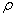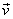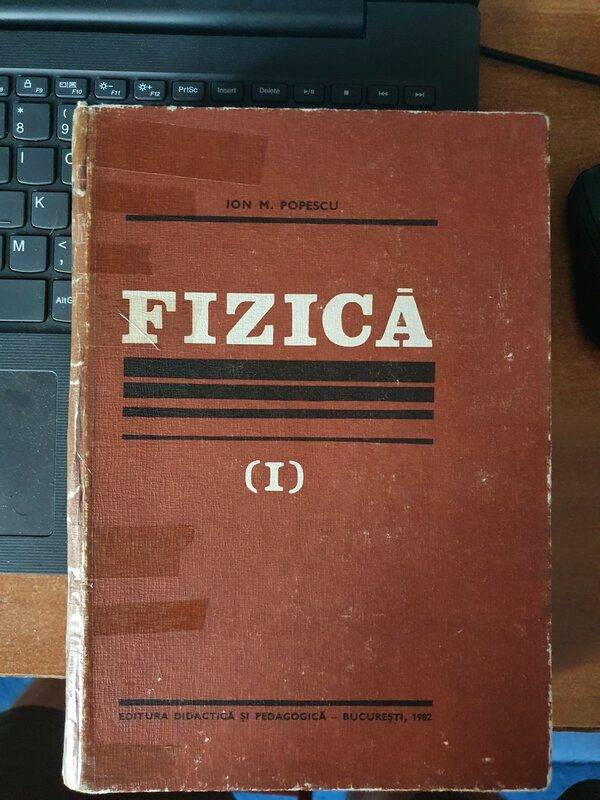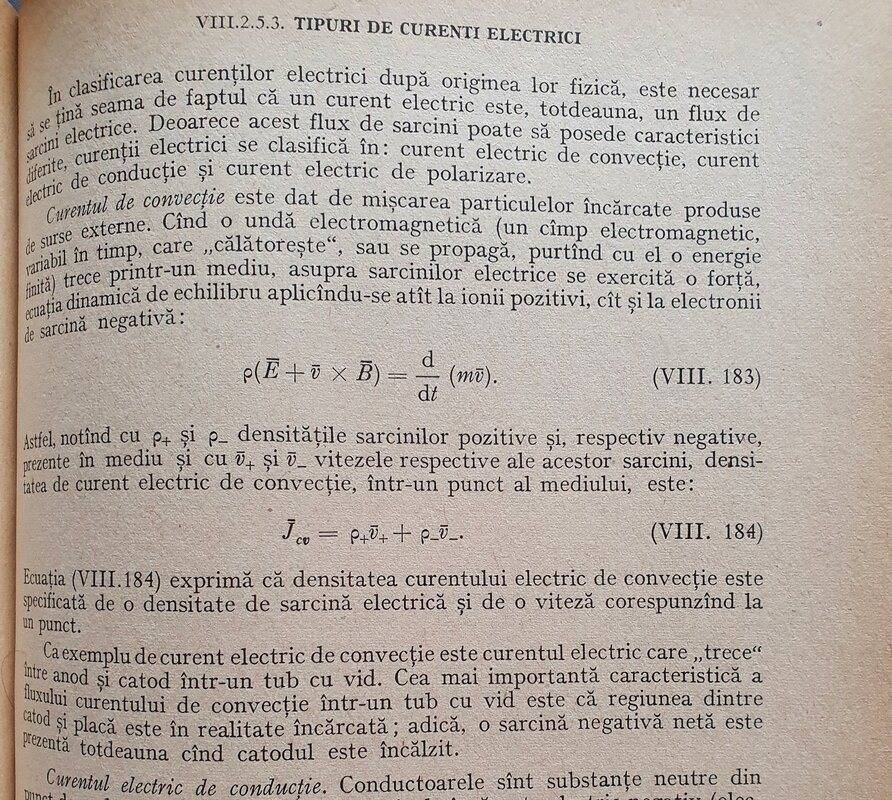# Convection current in electricity

• I
Summary:
So, I randomly came upon this in a textbook that I was skimming and I think I have a very very surface ideea of what it's about but I want to read and know more in order to understand the fenomenon in much more detail. Any help in any form would be much appreciated!

P.S.: This is my first post here, so yay!

Drakkith
Staff Emeritus
Interesting. This is the first time I've ever heard the term 'convection current' in the context of electricity and electrical charges. My very, very brief reading into the subject tells me that convection current is the movement of electrical charges in the absence of an electric field. One example is the movement of electrical charges in clouds due to air currents.

Also found: "convection current density is associated with beams of charged particles or motion of charged insulator,is the volume charge density andis its velocity"
From here: https://regi.tankonyvtar.hu/hu/tartalom/tamop425/0033_SCORM_GEFIT6102-EN/sco_02_01.scorm

And: "Convection current consists of charged particles moving in response to mechanical forces, as opposed to being guided by the electric field (Sections 2.2 and/or 5.1). An example of a convection current is a cloud bearing free electrons that moves through the atmosphere driven by wind."

•davenn and Baluncore
Baluncore
So, I randomly came upon this in a textbook that I was skimming and I think I have a very very surface ideea of what it's about but I want to read and know more in order to understand the fenomenon in much more detail.
Welcome to PF.
Perhaps you could identify the textbook and the subject being covered.

Where I first encountered this topic was in an old romanian textbook that I have called "Physics". The topic is part of a chapter called "Types of electric currents"
Here is section where they briefly touch on convection currentBaluncore
This section appears to be discussing the flow of space charge under the influence of an electric or magnetic field. It differentiates it from conduction in a metalic conductor. In English we do not use the term "convection current" in the same way.
TIPURI DE CURENTI ELECTRICI
TYPES OF ELECTRIC CURRENTS

In clasificarea curentilor electrici dupa originea lor fizica, este necesarsa se tina seama de faptul ca un curent electric este, totdeauna, un flux de sarcinii electrice.
In classifying electric currents according to their physical origin, it is necessary to take into account the fact that an electric current is always a flow of electric charge.

Deoarece acest flux de sarcini poate sa posede caracteristici diferite, curentii electrici se clasifica in; curent electric de convectie, current electric de conductie si curent electric de polarizare.
Because this flow of charges may have different characteristics, electric currents fall into; electric convection current, electric conduction current and polarization electric current.

Curentul de convectie este dat de miscarea particulelor incarcate produse de surse externe. Cind o unda electromagnetica (un cimp electromagnetic, variabil in timp, care "calatoreste", sau se propaga, purtind cu el o energie finita) trece printr-un mediu, asupra sarcinilor electrice se exercita o forta, ecuatia dinamica de echilibru aplicindu-se atit ionii pozitivi, cit si la electronii de sarcina negativa:
The convection current is given by the movement of charged particles produced by external sources. When an electromagnetic wave (an electromagnetic field, variable in time, which "travels", or propagates, carrying with it a finite energy) passes through an environment, a force is exerted on electric charges, the dynamic equation of equilibrium being applied both positive ions and negative charge electrons.

p( E + v X B ) = d/dt ( m v ). ( VIII. 183 )

Astfel, notind cu p+ si p- densitatile sarcinilor pozitive si, respectiv negative, prezente in mediu si cu v+ si v- vitezele respective ale acestor sarcini, densitatea de curent electric de convectie, intr-un punct al mediului, este;
Thus, noting with p+ and p- the densities of the positive and, respectively, negative charges present in the environment and with v+ and v- the respective velocities of these charges, the density of convection electric current, in a point of the environment, is;

Jcv = p+v+ + p-v- ( VIII. 184 )

Ecuatia (VIII. 184) experima ca densitatea curentului electric de convectie sete specificata de o densitate de sarcina electrica si de o viteza coresponzind la un punct.
Equation (VIII. 184) states that the density of the electric convection current is specified by an electric charge density and a velocity corresponding to a point.

Ca exemplu de curent electric de convectie este curentul electric care “trece” intre anod si catod intr-un tub cu vid.
An example of a convection electric current is the electric current that "passes" between the anode and the cathode in a vacuum tube.

Cea mai importanta caracteristica a fluxului curentului de convectie intr-un tub cu vid este ca regiunea dintre catod si placa este in realitate incarcata; adica, o sarcina negativa neta nete prezenta totdeauna cind catodul este incalzit.
The most important feature of the convection current flow in a vacuum tube is that the region between the cathode and the plate is actually charged; that is, a net negative charge is always present when the cathode is heated.

This section appears to be discussing the flow of space charge under the influence of an electric or magnetic field. It differentiates it from conduction in a metalic conductor. In English we do not use the term "convection current" in the same way.
Thanks for the answer, I'll follow the rabbit hole a little bit more.

What did you use to translate the stuff that were in the picture that I sent?

Baluncore